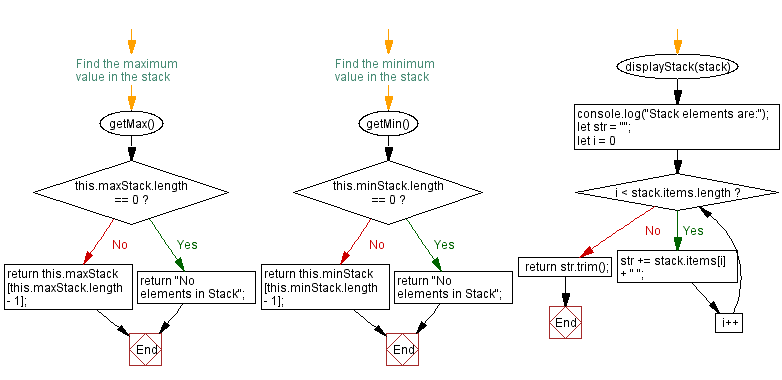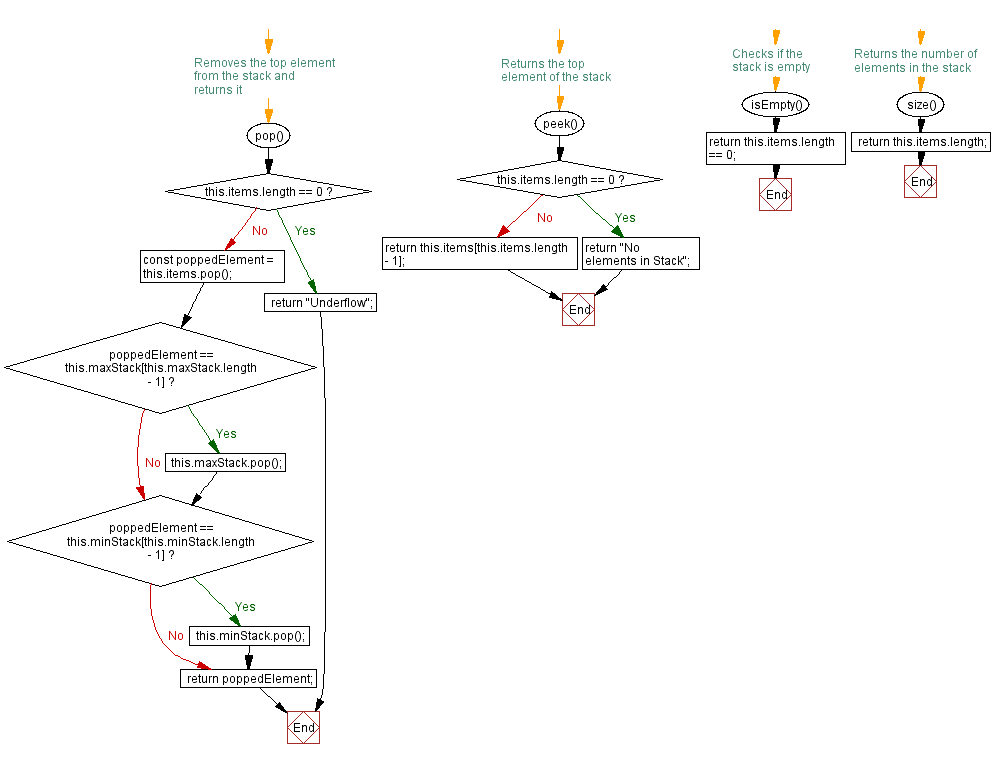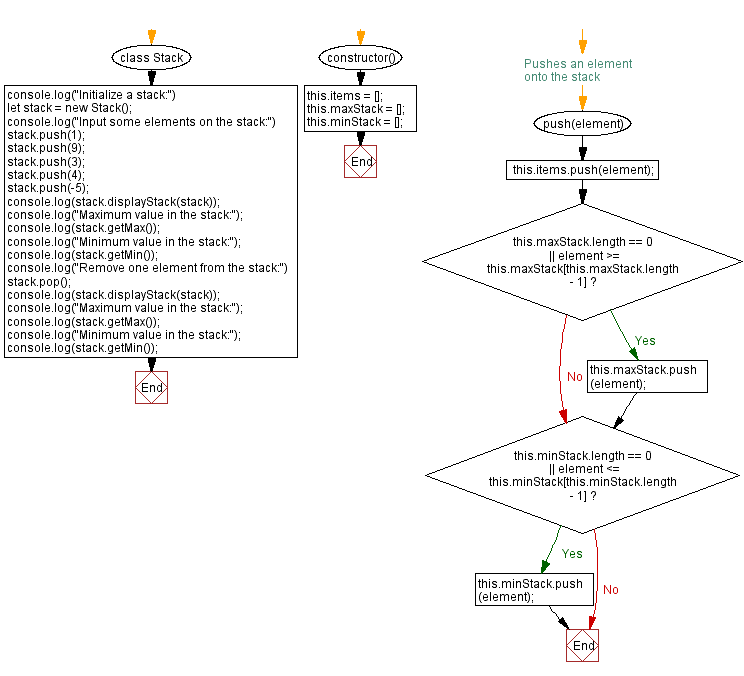# JavaScript Exercises: Find the maximum and minimum elements in a stack (using an array)

## JavaScript Stack: Exercise-5 with Solution

Write a JavaScript program to find the maximum and minimum elements in a stack.

Sample Solution:

JavaScript Code:

``````class Stack {
constructor() {
this.items = [];
this.maxStack = [];
this.minStack = [];
}

// Pushes an element onto the stack
push(element) {
this.items.push(element);

if (this.maxStack.length == 0 || element >= this.maxStack[this.maxStack.length - 1]) {
this.maxStack.push(element);
}

if (this.minStack.length == 0 || element <= this.minStack[this.minStack.length - 1]) {
this.minStack.push(element);
}
}

// Removes the top element from the stack and returns it
pop() {
if (this.items.length == 0)
return "Underflow";

const poppedElement = this.items.pop();

if (poppedElement == this.maxStack[this.maxStack.length - 1]) {
this.maxStack.pop();
}

if (poppedElement == this.minStack[this.minStack.length - 1]) {
this.minStack.pop();
}

return poppedElement;
}

// Returns the top element of the stack
peek() {
if (this.items.length == 0)
return "No elements in Stack";
return this.items[this.items.length - 1];
}

// Checks if the stack is empty
isEmpty() {
return this.items.length == 0;
}

// Returns the number of elements in the stack
size() {
return this.items.length;
}

// Find the maximum value in the stack
getMax() {
if (this.maxStack.length == 0)
return "No elements in Stack";
return this.maxStack[this.maxStack.length - 1];
}

// Find the minimum value in the stack
getMin() {
if (this.minStack.length == 0)
return "No elements in Stack";
return this.minStack[this.minStack.length - 1];
}

displayStack(stack) {
console.log("Stack elements are:");
let str = "";
for (let i = 0; i < stack.items.length; i++)
str += stack.items[i] + " ";
return str.trim();
}
}

console.log("Initialize a stack:")
let stack = new Stack();
console.log("Input some elements on the stack:")
stack.push(1);
stack.push(9);
stack.push(3);
stack.push(4);
stack.push(-5);
console.log(stack.displayStack(stack));
console.log("Maximum value in the stack:");
console.log(stack.getMax());
console.log("Minimum value in the stack:");
console.log(stack.getMin());
console.log("Remove one element from the stack:")
stack.pop();
console.log(stack.displayStack(stack));
console.log("Maximum value in the stack:");
console.log(stack.getMax());
console.log("Minimum value in the stack:");
console.log(stack.getMin());
```
```

Sample Output:

```Initialize a stack:
Input some elements on the stack:
Stack elements are:
1 9 3 4 -5
Maximum value in the stack:
9
Minimum value in the stack:
-5
Remove one element from the stack:
Stack elements are:
1 9 3 4
Maximum value in the stack:
9
Minimum value in the stack:
1
```

Flowchart:Live Demo:

See the Pen javascript-stack-exercise-5 by w3resource (@w3resource) on CodePen.

Improve this sample solution and post your code through Disqus

Stack Previous: Reverse the elements of a stack.
Stack Exercises Next: Remove all the elements from a given stack.

What is the difficulty level of this exercise?

Test your Programming skills with w3resource's quiz.

﻿